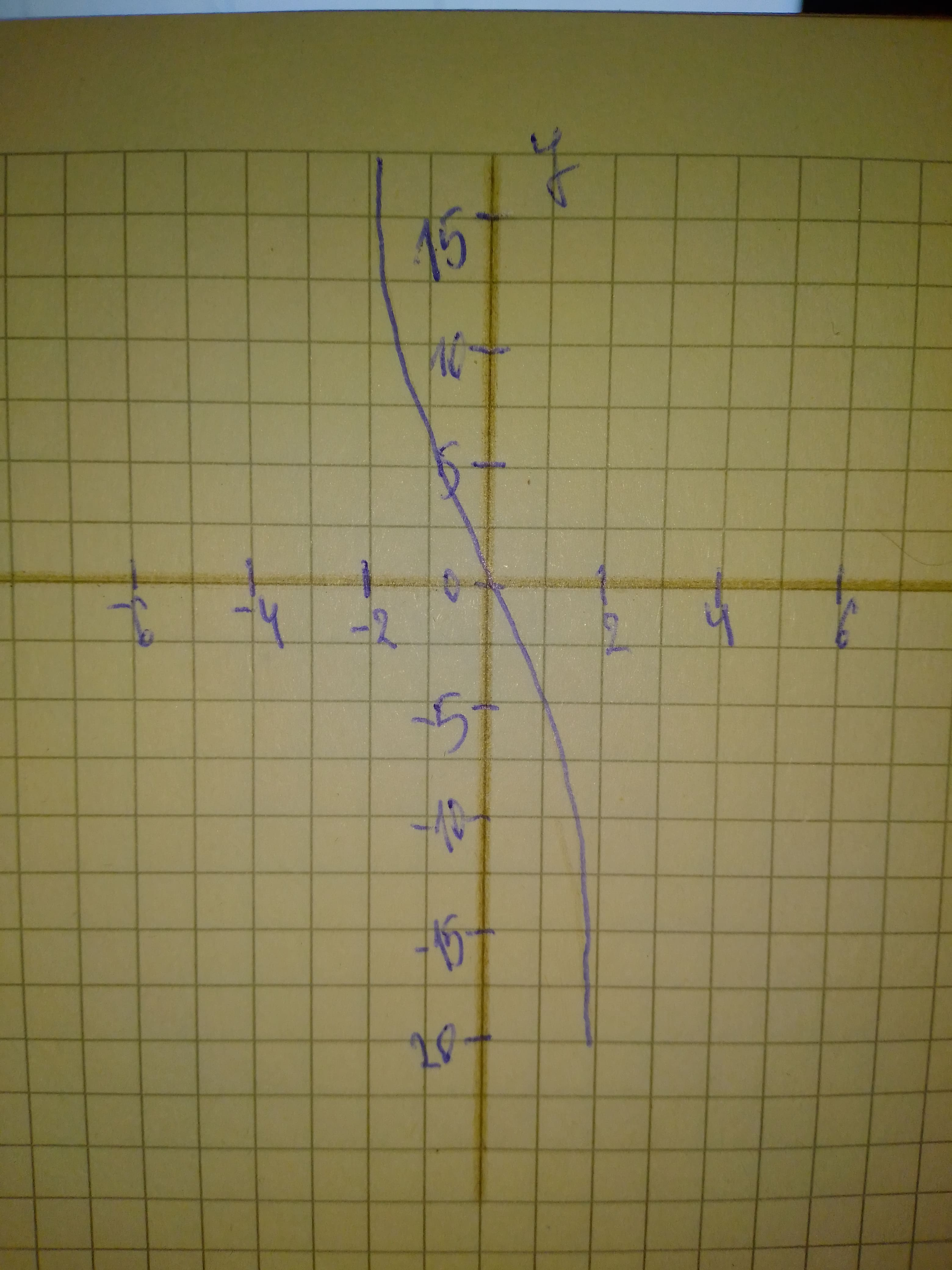# Use your knowledge of the graphs of polynomial functions to make a rough sketch of the graph of y=-2x^{3}+x^{2}-5x+2sanuluy 2021-01-06 Answered
Use your knowledge of the graphs of polynomial functions to make a rough sketch of the graph of $y=-2{x}^{3}+{x}^{2}-5x+2$
You can still ask an expert for help

• Questions are typically answered in as fast as 30 minutes

Solve your problem for the price of one coffee

• Math expert for every subject
• Pay only if we can solve itStrycharzT

Step 1
The expression is given as
$y=-2{x}^{3}+{x}^{2}-5x+2$
Step 2...........................